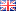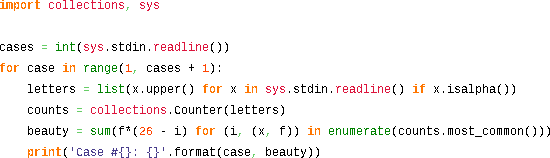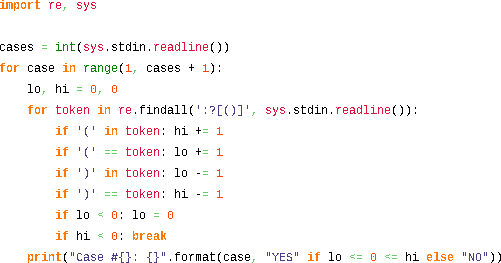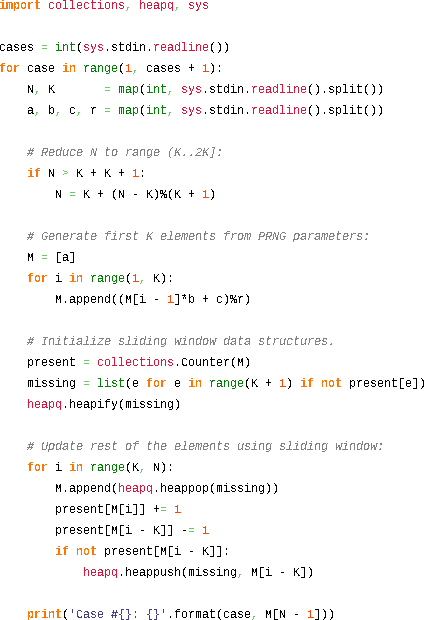## Facebook Hacker Cup 2013: qualification round problem analysisAs in previous years, I will be competing in the Facebook Hacker Cup, and I will describe the solutions I come up with on this weblog, hoping that other programmers or fellow competitors find them interesting.

I try to balance brevity with rigor: pasting just my solution code would not be very informative, but detailed proofs get boring quickly. Aiming for a happy medium, I will describe my solution approach before presenting the corresponding source code, adding proof outlines where necessary and linking to Wikipedia for detailed explanations of well-known topics.

This post contains source code written in Python. Unfortunately, Tweakers.net persists in their failure to support syntax highlighting for this popular language, which is why you will see screen shots below (but don't worry: links to the raw source code are provided as well).

#### Problem A: Beautiful Strings (20 points)

(Full problem statement here.)

We are asked to maximize the total “beauty” of a string, calculated as the sum of the beauty of the letters in the string, by assigning optimal values to different letters. The intuitive approach is to greedily assign the highest value (26) to the most common letter, the next highest value (25) to the next most common letter, and so on. Before coding this up, let's try to prove that the intuition is correct.

Formally, if we call value(x) the assigned value of letter x, and count(x) the number of times it occurs in the input string, then the total beauty equals the sum of count(x) × value(x) for all x, and we claim that a valuation is optimal if (and only if): value(x) > value(y) if count(x) > count(y).

This condition is necessary, because if value(x) > value(y) while count(x) < count(y), then swapping the values would increase the total beauty by (value(x) - value(y)) × (count(y) - count(x)) and therefore such a valuation cannot be optimal. The condition is also sufficient, because exchanging values for letters which occur equally often does not change the total beauty.

Now that we have proven the greedy approach to be correct, we can implement it in Python as follows:#### Problem B: Balanced Smileys (35 points)

(Full problem statement here.)

If we ignore the smileys for a moment, the problem reduces to checking if all parentheses in the input are properly balanced. We can check this in linear time by scanning the string once (e.g. from left to right) and tracking the current nesting depth, which is increased for every opening parenthesis we encounter, and decreased for every closing parenthesis.

Using this approach, the string is well-formed if and only if:
1. we end at nesting depth 0, and
2. the nesting depth never drops below 0.
For example, this is a string with balanced parentheses:
 Input text: Nesting depth: a ( b ( c ) d ( e ) ) f ( g ) h 0 0 1 1 2 2 1 1 2 2 1 0 0 1 1 0 0

But this string has an unmatched opening parenthesis, and thus violates rule 1:
 Input text: Nesting depth: a ( b ( c ) d 0 0 1 1 2 2 1 1

And this string has an unmatched closing parethesis, which violates rule 2:
 Input text: Nesting depth: a ) b ( c 0 0 -1 -1 0 0

This approach works well with just parentheses, but the presence of smileys complicates matters, because we don't know in advance if we should count them as parentheses or not. Fortunately, we can adapt the above algorithm to deal with this uncertainty. Instead of tracking a single nesting depth value at each position, we should keep track of a set of integers representing all possible nesting depths.

Since this set will necessarily consist of consecutive integers, we can just store the minimum and maximum elements (knowing that all values in between are possible too). Again, we conclude that the string is well-formed if the lower-bound at the end is 0, and the upper bound never becomes negative (which would imply the set of possibilities is empty).

For example, this string is well-formed:

 Input text: Lower bound: Upper bound: ( ( : ) : ) ) 0 1 2 1 0 0 0 1 2 2 2 1

This idea can be implemented succinctly in Python:Note that this solution asymptotically optimal: it requires linear time and constant space.

#### Problem C: Find The Min (45 points)

(Full problem statement here.)

The final problem looks complicated, with all the parameters and formulas described in the problem statement, but we can approach it systematically by breaking it down into simpler subproblems.

First, the problem statement dictates that the input is generated using a pseudo-random linear congruential generator. This is only done to keep the size of the input files small, so we can generate the first K elements of the array using the the provided formula, and then forget about the RNG parameters for the rest of the problem.

Although these first K values could be anything, we can make some useful observations about the contents of the array after the initial K elements:
1. Every element will be between 0 and K (inclusive) by the pigeonhole principle.
2. Consequently, every window of K + 1 consecutive elements will contain each value between 0 and K exactly once (i.e. it contains a permutation of the integers 0 through K).
3. Consequently, for i > 2K: M[i] = M[i - (K + 1)].
The final conclusion is useful because it implies that the generated array is cyclic with period K + 1. Below is a simple example with K = 4, N = 18, where this is property is clear:
 Index Value 0 1 2 3 4 5 6 7 8 9 10 11 12 13 14 15 16 17 3 1 4 1 0 2 3 4 1 0 2 3 4 1 0 2 3 4

This means that if we can compute the elements at indices K through 2K (inclusive), we have effectively computed them all. K is not ridiculously large (at most 100,000) but we should still be somewhat efficient in our implementation. I used a sliding window algorithm in which the array is calculated from left to right, while two data structures are maintained that contain information about the preceding K elements which is used to quickly calculated new elements.

The first data structure counts how often each distinct value is present in the window of K preceding elements. This could be a simple array of K+1 integers (though I found Python's Counter class slightly more convenient).

The second data structure is an ordered collection of integers (between 0 and K, inclusive) that are missing in the same window. Of course, I want to take the minimum element from this list at each step, and I want to be able to update it efficiently. Therefore, a plain list isn't the right choice. Instead, I will use a heap structure, although an ordered binary search tree (like Java's TreeSet or C++'s std::set) would also be appropriate.

Note that the present and missing data structures complement each other: if a value is stored in missing, then its count in present will be zero. And vice versa: if a value is not in missing then it must appear in the current window, and its count in present will be nonzero

Now consider how these data structures are updated when the window slides to the right. First, to determine M[i] for an index i ≥ K, I can remove the lowest value from the missing set, and then increment present\[M[i]], thus extending the window on the right by one element. To shrink the window on the left, I need to decrement present\[M[i - K]]. If the resulting count has reached zero, that means M[i - K] doesn't occur anywhere else in the search window, and it should be added to missing.

The implementation in Python looks like this:Since heap operations on a list of size O(K) take O(log K) time, this algorithm runs in O(K×log K) time and O(K) space. Although this is fast enough for this contest, I suspect this is not optimal, and O(K) time should be possible too. If you know how to do it, please leave a comment describing your approach!